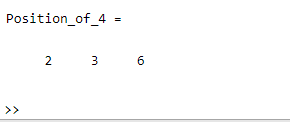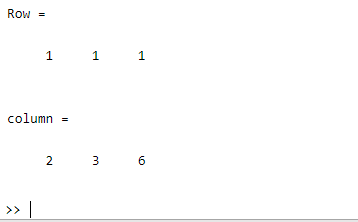Related Articles

# How to Find the Position of a Number in an Array in MATLAB?

• Last Updated : 04 Jul, 2021

Finding the position of a number in an array, which can be done using the find() function. The find() function is used to find the indices and values of the specified nonzero elements.

### Syntax

`find(X)`

Parameters: This function accepts a parameter.

• X: This is the specified number whose position is going to be found in the array.

Return Value: It returns the position of the given number in a specified array.

Example 1

## Matlab

 `% MATLAB code for getting the position ``% of element 4``X = [2 4 4 5 6 4]; ``% Initializing an array ``% of some elements`` ` `% Calling the find() function to find ``% the position of 4``Position_of_4 = find(X==4)`

Output:Example 2

## Matlab

 `% MATLAB code for getting the position ``% of element 4 in terms of rows and columns.``X = [2 4 4 5 6 4]; `` ` `% Calling the find() function to find ``% the position of 4 in terms of rows ``% and columns``[Row, column] = find(X==4)`

Output:My Personal Notes arrow_drop_up